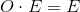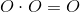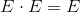## Example Questions

### Example Question #102 : Integers

Ifandare both odd integers, which of the following is not necessarily odd?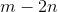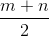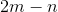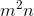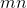Explanation:

With many questions like this, it might be easier to plug in numbers rather than dealing with theoretical variables.  However, given that this question asks for the expression that is not always even or odd but only not necessarily odd, the theoretical route might be our only choice.

Therefore, our best approach is to simply analyze each answer choice.:  Sinceis odd,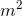is also odd, since and odd number multiplied by an odd number yields an odd product.  Sinceis also odd, multiplying it bywill again yield an odd product, so this expression is always odd.:  Sinceis odd, multiplying it by 2 will yield an even number.  Subtracting this number fromwill also give an odd result, since an odd number minus an even number gives an odd number.  Therefore, this answer is also always odd.:  Since both numbers are odd, their product will also always be odd.:  Sinceis odd, multiplying it by 2 will give an even number.  Sinceis odd, subtracting it from our even number will give an odd number, since an even number minus and odd number is always odd.  Therefore, this answer will always be odd.:   Since both numbers are odd, there sum will be even.  However, dividing an even number by another even number (2 in our case) does not always produce an even or an odd number.  For example, 5 and 7 are both odd.  Their sum, 12, is even.  Dividing by 2 gives 6, an even number.  However, 5 and 9 are also both odd.  Their sum, 14, is even, but dividing by 2 gives 7, an odd number.  Therefore, this expression isn't necessarily always odd or always even, and is therefore our answer.

### Example Question #103 : Integers

Theodore hasjelly beans. Portia has three times that amount. Harvey has five times as many as she does. What is the total count of jelly beans in the whole group?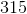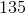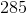Explanation:

To find the answer to this question, calculate the total jelly beans for each person:

Portia:* <Theodore's count of jelly beans>, which is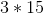orHarvey:* <Portia's count of jelly beans>, which is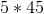or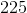So, the total is: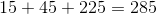(Do not forget that you need those originalfor Theodore!)

### Example Question #3 : Even / Odd Numbers

Find the product ofand.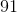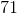Explanation:

Rewrite the product into the expression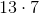, and multiply the ones digit of both numbers.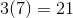The ones digit of the final answer is the ones digit from the multiplication of 3 and 7 thus the 1.  The tens digit, 2, will be carried over to the next calculation.

Multiply the tens digit of 13 to 7 and add the carry over.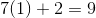The value of 9 is the tens digit of the final answer.

Combine the tens digit with the ones digit.  The answer is.

### Example Question #11 : Even / Odd Numbers

The product of two numbers is a negative odd integer. Which statement must be true about the numbers?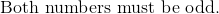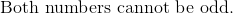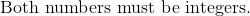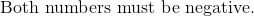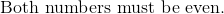Explanation:

For the product of two numbers to be even, one number must be even. For the product of two numbers to be odd, both numbers must be odd.

Remember: